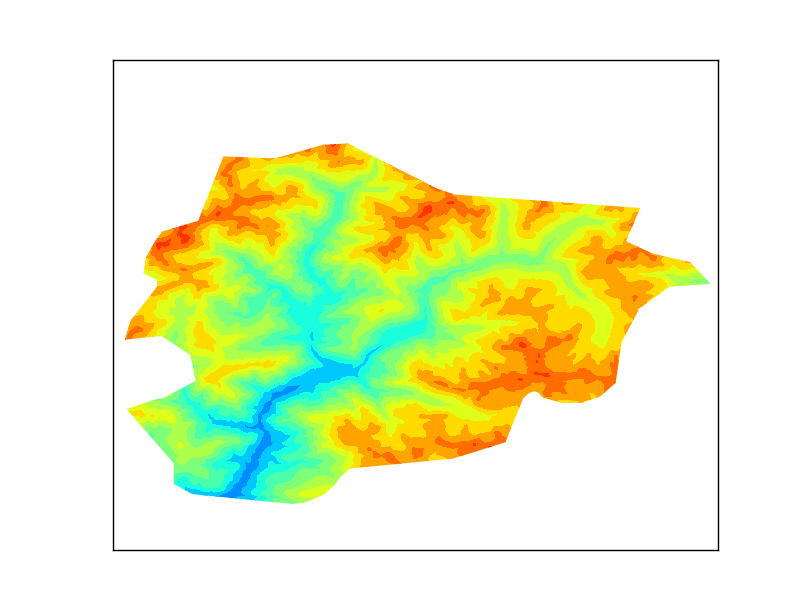## Tuesday, May 26, 2015

### The blog has moved!

After four years hosted at blogger, I decided to move the blog to its own domain:

http://geoexamples.com is here!

The new blog uses Jekyll, so the interactive will work much better.

If you are using the feedburner rss, the feed will continue at the same place, and the old posts won't be erased.

## Tuesday, May 12, 2015

### d3-composite-projections

Some countries have regions sparse around the globe, which adds difficulties when drawing maps for them.
D3 already had the albersUsa projection that solved this problem by creating a composed projection, moving Alaska and Hawaii close to the main part of the USA.
But the other countries didn't have a projectino like this. That's why I made this library.

It adds the composite projection for:
With a function that draws a border between the composition zones by returning an SVG path.

There is an example for each region, linked in the list above.

The library web page explains the usage and  installation/testing

## Monday, March 30, 2015

### D3js mapping presentation at Girona

Every year, SIGTE organizes workshops and a conference about Free GIS software in Girona.

This year I gave a workshop about D3js mapping.
The slides (in Spanish) are here: http://rveciana.github.io/Mapas-web-interactivos-con-D3js/
The examples can be found at my bl.ocks.org space: http://bl.ocks.org/rveciana named with the prefix JSL 2015.

## Wednesday, November 26, 2014

### Basemap Tutorial

Basemap is a great tool for creating maps using python in a simple way. It's a matplotlib <http://matplotlib.org/>_ extension, so it has got all its features to create data visualizations, and adds the geographical projections and some datasets to be able to plot coast lines, countries, and so on directly from the library.

Basemap has got some documentation <http://matplotlib.org/basemap/index.html>_, but some things are a bit more difficult to find. I started a readthedocs page to extend a little the original documentation and examples, but it grew a little, and now covers many of the basemap possibilities.Some of the examples from the tutorial

The tutorial can be found at http://basemaptutorial.readthedocs.org/, and all the examples and its source code, at GitHub and it's available for sharing or being modified by adding the attribution.

The tutorial covers:
I would really appreciate some feedback, the comments are open!

## Saturday, October 11, 2014

### Basemap raster clipping with a shapefile

Basemap is a great library for mapping faster than other python options, but there are some usual things I couldn't find how to do. Clipping a raster using a shape is one of them. Here's how do I do itThe output
As usual, all the code can be found at GitHub

### Getting some data

The example plots some elevation data, taken from the SRTM. After looking for some options, the easiest to work with was this one: http://srtm.csi.cgiar.org/SELECTION/inputCoord.asp
The shapefile will be the border of Andorra, taken from Natural Earth
The result is a little poor because the resolution is low, but works well for the example.

### The script

from mpl_toolkits.basemap import Basemap
from matplotlib.path import Path
from matplotlib.patches import PathPatch
import matplotlib.pyplot as plt
from osgeo import gdal
import numpy
import shapefile

fig = plt.figure()

for shape_rec in sf.shapeRecords():
if shape_rec.record == 'Andorra':
vertices = []
codes = []
pts = shape_rec.shape.points
prt = list(shape_rec.shape.parts) + [len(pts)]
for i in range(len(prt) - 1):
for j in range(prt[i], prt[i+1]):
vertices.append((pts[j], pts[j]))
codes += [Path.MOVETO]
codes += [Path.LINETO] * (prt[i+1] - prt[i] -2)
codes += [Path.CLOSEPOLY]
clip = Path(vertices, codes)
clip = PathPatch(clip, transform=ax.transData)

m = Basemap(llcrnrlon=1.4,
llcrnrlat=42.4,
urcrnrlon=1.77,
urcrnrlat=42.7,
resolution = None,
projection = 'cyl')

ds = gdal.Open('srtm_37_04.tif')

gt = ds.GetGeoTransform()
x = numpy.linspace(gt, gt + gt * data.shape, data.shape)
y = numpy.linspace(gt, gt + gt * data.shape, data.shape)

xx, yy = numpy.meshgrid(x, y)

cs = m.contourf(xx,yy,data,range(0, 3600, 200))

for contour in cs.collections:
contour.set_clip_path(clip)

plt.show()
• I used the pyshp library for reading the shapefile, since Fiona and GDAL don't work well together, and OGR was longer
• Lines 14 to 27 create the path. A Matplotlib path is made by two arrays. One with the points (called vertices in the script), and the other with the functions for every point (called codes)
• In our case, only straight lines have to be used, so there will be a MOVETO to indicate the beginning of the polygon, many LINETO to create the segments and one CLOSEPOLY for closing it
• Of course, only the polygon for Andorra has to be used. I get it from the shapefile attributes
•  The prt array is for managing multipolygons, which is not the case, but the code will create correct clipping for multipolygons
• The path is created using the Path function, and then added to a PathPatch, to be able to use it as a closed polygon. Note the trasnform=ax.transData attribute. This assumes the polygon coordinates to be the ones used in the data (longitudes and latitudes in our case). More information here
• Next code lines draw the map as usual. I have used a latlon projection, so all the values for the raster and shapefile can be used directly. If the output raster was in an other projection, the shapefile coordinates should be appended to the path using the output projection (m(pts[j], pts[j]))
• The x and y coordinates are calculated from the GDAL geotransform, and then turned into a matrix using meshgrid
• The clipping itself is made in the lines 48 and 49. For each drawn element, the method set_clip_path is applied